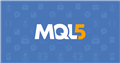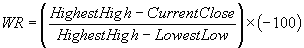# iWPR Reference Points

Hi all, just after a bit of clarity on the use of iWPR.

When using the iRSI function it has four parameters, with the last being ENUM_APPLIED_PRICE, so if for example I use PERIOD_M15 and set the final parameter to PRICE_CLOSE, create an array and then reference it, i.e rsiVAL, I am getting the closing RSI value from the second last candle (30mins ago).

However, iWPR does not have this fourth parameter, so where is the WPR value coming from? Using the same example array and trying to get the wprVAL, what value will be returned? Obviously it will still reference the PERIOD_M15 but is it still the PRICE_CLOSE? I would assume it would be but the iWPR reference page isn't clear (to me at least). Is there a default price type if not explicitly listed?

TIADocumentation on MQL5: Technical Indicators / iWPR
• www.mql5.com
iWPR - Technical Indicators - MQL5 Reference - Reference on algorithmic/automated trading language for MetaTrader 5

JimboDiggity: Hi all, just after a bit of clarity on the use of iWPR. When using the iRSI function it has four parameters, with the last being ENUM_APPLIED_PRICE, so if for example I use PERIOD_M15 and set the final parameter to PRICE_CLOSE, create an array and then reference it, i.e rsiVAL, I am getting the closing RSI value from the second last candle (30mins ago). However, iWPR does not have this fourth parameter, so where is the WPR value coming from? Using the same example array and trying to get the wprVAL, what value will be returned? Obviously it will still reference the PERIOD_M15 but is it still the PRICE_CLOSE? I would assume it would be but the iWPR reference page isn't clear (to me at least). Is there a default price type if not explicitly listed? TIA

iWPR does not have an applied price parameter because Larry Williams' Percent Range indicator depends on multiple price quotes and not just one.## Calculation

Below is the formula of the %R indicator calculation, which is very similar to the Stochastic Oscillator formula:

%R = -(MAX (HIGH (i - n)) - CLOSE (i)) / (MAX (HIGH (i - n)) - MIN (LOW (i - n))) * 100

Where:

CLOSE (i) — today's closing price;
MAX (HIGH (i - n)) — the highest maximum over a number (n) of previous periods;
MIN (LOW (i - n)) — the lowest minimum over a number (n) of previous periods.

Fernando Carreiro #:

iWPR does not have an applied price parameter because Larry Williams' Percent Range indicator depends on multiple price quotes and not just one.

## Calculation

Below is the formula of the %R indicator calculation, which is very similar to the Stochastic Oscillator formula:

%R = -(MAX (HIGH (i - n)) - CLOSE (i)) / (MAX (HIGH (i - n)) - MIN (LOW (i - n))) * 100

Where:

CLOSE (i) — today's closing price;
MAX (HIGH (i - n)) — the highest maximum over a number (n) of previous periods;
MIN (LOW (i - n)) — the lowest minimum over a number (n) of previous periods.

Hi Fernando thanks for your response. Going off what you said does that mean I am incorrectly using the iRSI also, in that the ENUM_APPLIED_PRICE determines the prices used in the calculation of the RSI and not what value the RSI was at a specific point in time? I.e. I thought using PRICE_OPEN would give me the RSI value at the open of the candle, but I'm now assuming it will always give me the value at the close of the candle, but uses the opening prices in its calculation? Does that make sense? Having thought about it, it seems logical to be honest..

Further, and to be clear, does this mean that using iWPR will always output the closing WPR value (in my example the value at the close of the second previous bar)?

JimboDiggity #: Hi Fernando thanks for your response. Going off what you said does that mean I am incorrectly using the iRSI also, in that the ENUM_APPLIED_PRICE determines the prices used in the calculation of the RSI and not what value the RSI was at a specific point in time? I.e. I thought using PRICE_OPEN would give me the RSI value at the open of the candle, but I'm now assuming it will always give me the value at the close of the candle, but uses the opening prices in its calculation? Does that make sense? Having thought about it, it seems logical to be honest..

Further, and to be clear, does this mean that using iWPR will always output the closing WPR value (in my example the value at the close of the second previous bar)?

You are mixing apples and oranges! If RSI has an applied price parameter, then it uses the applied price for its calculations.

If you select open price, then it will use the open price and the RSI will not change over the entire lifetime of the bar, because that RSI was calculated for that bar's opening price.

Fernando Carreiro #:

You are mixing apples and oranges! If RSI has an applied price parameter, then it uses the applied price for its calculations.

If you select open price, then it will use the open price and the RSI will not change over the entire lifetime of the bar, because that RSI was calculated for that bar's opening price.

Got it, but as to my second point and clarity specifically for when referencing the values in an array, if i look back at the WPR (or RSI) value from 2 candles ago, it will ALWAYS provide the value present at the END of the candle (even if it was calculated at the start, and unchanged throughout the life of the candle), correct?

JimboDiggity #: Got it, but as to my second point and clarity specifically for when referencing the values in an array, if i look back at the WPR (or RSI) value from 2 candles ago, it will ALWAYS provide the value present at the END of the candle (even if it was calculated at the start, and unchanged throughout the life of the candle), correct?

I have already answered but I will try explain it a different way.

RSI and WPR calculations on past bars are not dependant on "time"—they are dependant on "price". When looking at bars that have already closed (not the current bar), then it does not matter when the calculations are done, because the source of the calculation, namely the open-high-low-close prices will no longer change.

Now, when looking at the current bar, things change a bit, not because of "time", but still because of "price"—the close price is still changing, which can still affect the high and low prices, but not the open price.

So, if you use RSI of open price, then it will NEVER change, not even for the current bar.

As for WPR, it too will never change for past bars. It will only change for the current bar, because it is dependant on the close, high and low.

Fernando Carreiro #:

I have already answered but I will try explain it a different way.

RSI and WPR calculations on past bars are not dependant on "time"—they are dependant on "price". When looking at bars that have already closed (not the current bar), then it does not matter when the calculations are done, because the source of the calculation, namely the open-high-low-close prices will no longer change.

Now, when looking at the current bar, things change a bit, not because of "time", but still because of "price"—the close price is still changing, which can still affect the high and low prices, but not the open price.

So, if you use RSI of open price, then it will NEVER change, not even for the current bar.

As for WPR, it too will never change for past bars. It will only change for the current bar, because it is dependant on the close, high and low.

Yeah so i think there was likely some minor misinterpretations going on but essentially we believe the same (after your first post). Thanks for your help and clarification!

JimboDiggity #: Yeah so i think there was likely some minor misinterpretations going on but essentially we believe the same (after your first post). Thanks for your help and clarification!

You are welcome! However, "belief" has nothing to do with it!

Either you fully understand something or you don't. Even a minor misinterpretation can cause a major issue.

So, dedicate some time and experimentation to making sure you fully understand it.

Reason: